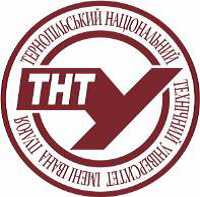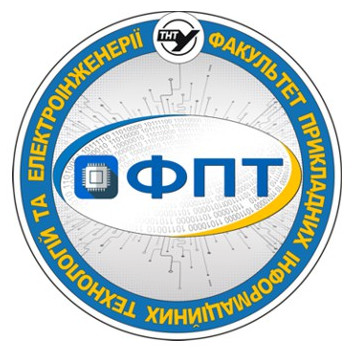## SyllabusTernopil Ivan Puluj National Technical UniversityКаф. вищої математики

Higher Mathematics

syllabus

## 1. Educational programs for which discipline is mandatory:

# Educational stage Broad field Major Educational program Course(s) Semester(s)
1 bachelor's 13. Механічна інженерія 131. Прикладна механіка (бакалавр) 1 1-2
2 bachelor's 19. Архітектура та будівництво 192. Будівництво та цивільна інженерія (бакалавр) 1 1-2
3 bachelor's 14. Електрична інженерія 141. Електроенергетика, електротехніка та електромеханіка (бакалавр) 1 1-2
4 bachelor's 15. Автоматизація та приладобудування 151. Автоматизація та комп’ютерно-інтегровані технології (бакалавр) 1 1-2

## 3. Information about the author of the course

Full name Leonid Romaniuk
Link to the teacher`s page on the official website of the University http://library.tntu.edu.ua/personaliji/a/r/romanjuk-leonid-antonovych/
Е-mail (in the domain tntu.edu.ua)

## 4. Information about the course

Study hours structure Lectures: 86
Practical classes: 86
Laboratory classes: 0

Amount of hours for individual work: 218
ECTS credits: 13
Teaching language english
Form of final examination credit
exam
Link to an electronic course on the e-learning platform of the university https://dl.tntu.edu.ua/bounce.php?course=4839

## 5. Program of discipline

### Description of academic discipline, its goals, subject of study and learning outcomes

The purpose of the study of the discipline "Higher Mathematics" is the formation of a system of theoretical and practical knowledge on linear algebra, analytic geometry, mathematical analysis, differential equations and special issues of mathematics.
The main task of studying the discipline is to master students with the necessary mathematical apparatus, which helps to teach mathematical models of processes and structures associated with the subsequent activities of specialists, to study such models, interpret the results accordingly.

### The place of academic discipline in the structural and logical scheme of study according to the educational program

#### Prerequisites. List of disciplines, or knowledge and skills, possession of which students needed (training requirements) for successful discipline assimilation

Possession of an elementary level of knowledge from the school course of mathematics.

#### List of disciplines based on learning results from this discipline

Probability Theory and Mathematical Statistics
High-Voltage Engineering Equipment
Mathematical problems in electrical power engineering

### Contents of the academic discipline

#### Lectures (titles/topics)

1. Matrices and Matrix operations.
2. Determinant of a matrix.
3. Solving System of linear equations.
4. Solving System of linear equations by Gauss Elimination.
5. Homogenous systems of equations.
6. Vectors. Linear operations under vectors, their properties.
7. Basis of vectors, the components of the vector.
8. Scalar, vector, triple scalar products.
9. A straight line in the plane.
10. The curves of the second degree.
11. A plane in the space.
12. A straight line in the space.
13. Functions: essentials definitions.
14. Number sequences. The limit of the number sequence.
15. The limit of the function.
16. Continuity.
17. The Definition of the Derivative.
18. Techniques of Differentiation.
19. The Differential of the function.
20. Basic Theorems of Calculus.
21. Applications of Derivative.
22. Functions of Several Variables.
23. Partial Differentiation.
24. The geometrical application of Partial Derivatives.
25. Extrema of Functions of Two Variables.
26. The factorization of polynomial.
27. Indefinite Integral. Basic Methods of Integration.
28. Integration of Rational Functions, the Method of Partial Fractions.
29. Integration of trigonometric functions.
30. Integration of irrational functions.
31. The Definite Integrals and their Properties.
32. Improper Integrals.
33. Applications of the Integral.
34. Infinite Series.
35. Alternating Series. Functional Series.
36. Power Series.
37. Taylor and Maclaurin Series.
38. Fourier series.

#### Practical classes (topics)

1. Linear operations on matrices. Matrix multiplication.
2. Evaluating the determinant using Laplace expansion theorem and properties of determinant.
3. Finding the inverse of the matrix. Solving the system using the inverse matrix.
4. Solving the system using Cramer’s rule, Gauss elimination method.
5. Solving the consistent undefined system, general solution. Gauss−Jordan reduction. Solving homogenous system.
6. Finding components of a vector, magnitude of a vector. Direction cosines. Linear operations in components form. Division Point of a line segment. Midpoint of a line segment.
7. Application of Scalar product, Vector product, Triple Scalar product for solving problems.
8. Using standard forms of the equation of a line in the plane for solving problems. Finding a distance from a point to a line, equations of Angle Bisectors.
9. Finding standard equations of conic curves.
10. Finding equations of a plane in the space. Determine the angle between two planes. Different forms of representation of a line in the space.
11. Finding the domain of a function. Investigation functions as even, odd, or neither. Elementary functions, their graphs and properties. Sketching the graphs of functions by using translation and reflection.
12. Sequences. The limit of sequence. Rules for convergence sequences. Techniques of finding Limits of a sequence and a function.
14. Continuity at a point. Continuity test. Finding points of discontinuity. One-Sided Continuity. Testing for continuity on an interval.
15. Techniques of Differentiation. Finding Derivative using the definition. Using the basic rules for finding derivatives. Finding an equation of a tangent line and a normal line to a graph.
16. Techniques of Differentiation. Using the chain rule for finding derivatives of the composite function. General procedure for implicit differentiation. Logarithmic Differentiation.
17. Applications of Derivatives. Find the extreme values of function. Monotonic functions and the first derivative test. First Derivative test for Local Extrema.
18. Find the Absolute Extrema of Continuous Function on a Finite Closed Interval. Differential and approximation. Higher-Order Differentials.
19. The Second Derivative Test for Concavity. Point of Inflection. Graphing a function.
20. Applications of Derivatives for the problems involving Maxima and Minima.
21. Finding the domain of a function of two variables. Level curves and the graph of function. Finding and evaluating a partial derivative, higher partial derivative.
22. The total differential. Application of differential for approximate computations.
23. Finding the Tangent Plane and Normal Line of the surface. Estimating Change in the Value of Function in a particular direction.
24. Finding the local extreme values of the function of two variables. Finding Absolute Extrema on Closed Bounded Regions.
25. Techniques of Integration. Basic Integration Formulas. Evaluating the Indefinite Integrals by using the Simple
Substitutions to reduce the integrals to standard form. More Complicated Substitutions. Integration by Parts.
26. Complex numbers and operations on them. Factoring of the polynomial on the multipliers.
27. Integration of Rational Functions by Partial Fractions. Finding undetermined coefficients.
28. Integration of trigonometric functions. Using Weierstrass Substitution.
29. Integration of irrational functions. Trigonometric Substitution for Radical functions. Quadratic-form integrals, Integration by completing the square. Integration of differential binomials.
30. Formula of Newton-Leibnitz. Evaluating Definite Integrals by The Fundamental Theorem. Integration by parts. Calculations of the definite integrals using the method of substitution.
31. Applications of the definite integral: calculation the areas of the figures, the volumes of the bodies, the length of the arc of the curve, the area of the surface of rotation.
32. Determining Convergence or Divergence of the series with positive terms.
33. Determining Absolute and Conditional Convergence of Alternating Series. Leibniz’s alternating series test. Finding the Functional series’ interval of convergence. Finding the Radius and the interval of Convergence of a Power Series.
34. Using series to approximate the values of the functions and the integrals.
35. Finding a Fourier Series Expansion of 2П– periodic function. The Calculation of the Fourier coefficients for an even and an odd function.
36. Expanding of 2l – periodic function into Fourier series. Fourier-series expansion of nonperiodic function.

### Learning materials and resources

1. Differential and Integral Calculus for One Variable Functions. / L.V. Kurpa, T.V. Smatko. Differential and Integral Calculus for One Variable Functions: Textbook. – Kharkiv: NTU KhPI: 2017. -324 pages.
2. Gilbert Strang. Introduction to Linear Algebra. Fifth Edition. (May 2016). ISBN 978-09802327-7-6
3.Gilbert Strang. Calculus. Second Edition. (August 2010).ISBN 978-09802327-4-5
4. Robert G. Bartle and Donald R. Sherbert, Introduction to real analysis, 3rd ed., John Wiley & Sons Inc., New York, 2000.
5. John P. D’Angelo and Douglas B. West, Mathematical Thinking: Problem-Solving and Proofs, 2nd ed., Prentice Hall, 1999.
6. Walter Rudin, Principles of mathematical analysis, 3rd ed., McGraw-Hill Book Co., New York, 1976. International Series in Pure and Applied Mathematics.

## 6. Policies and assessment process of the academic discipline

### Assessment methods and rating system of learning results assessment

As a result of studying a student, a student must demonstrate the following learning outcomes:
- Ability to apply elements of linear algebra and analytic geometry;
- Ability to apply elements of mathematical analysis;
- Ability to apply differential calculus;
- Ability to apply integral calculus.

Table of assessment scores:

 Assessment scale VNZ(100 points) National(4 points) ECTS 90-100 Excellent А 82-89 Good B 75-81 C 67-74 Fair D 60-66 E 35-59 Poor FX 1-34 F
Approved by the department
(protocol №
on «
»
y.).